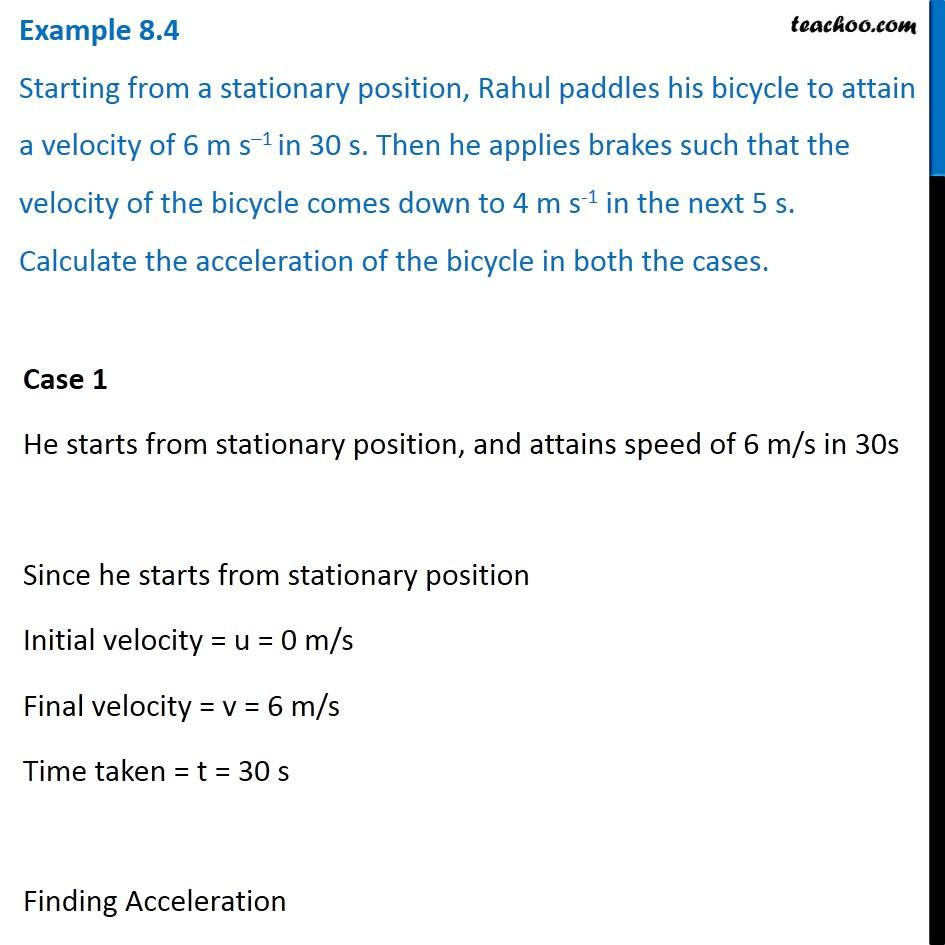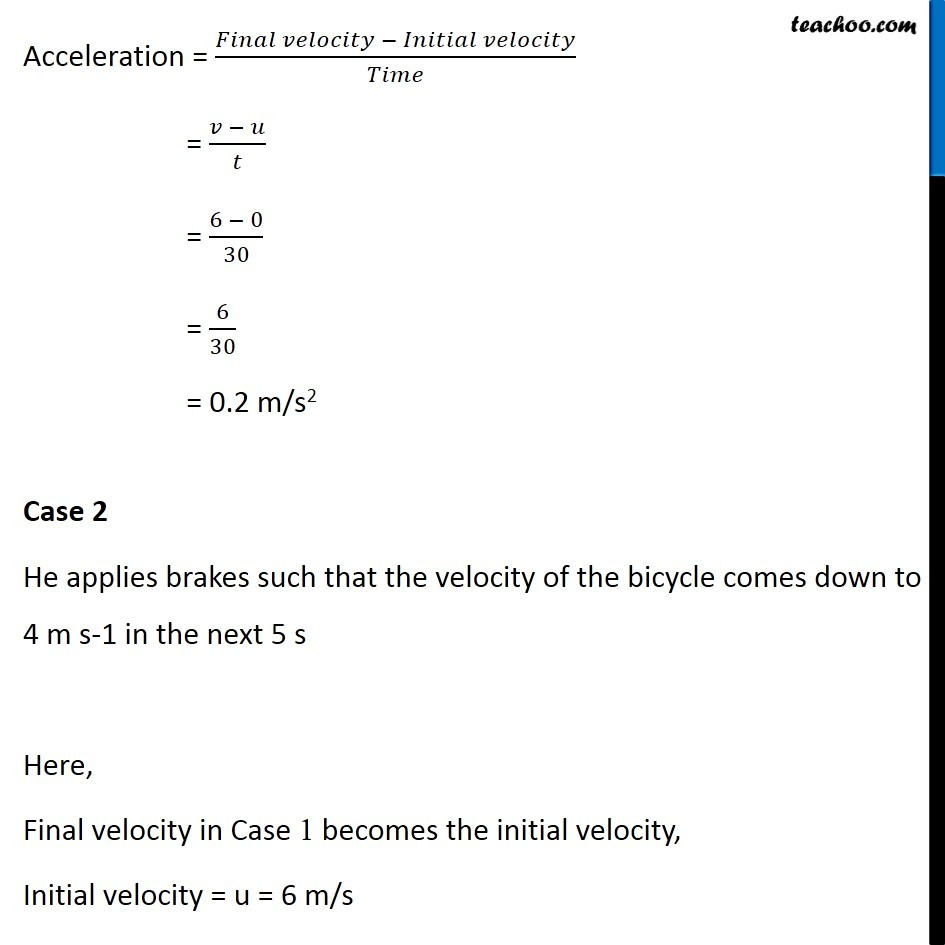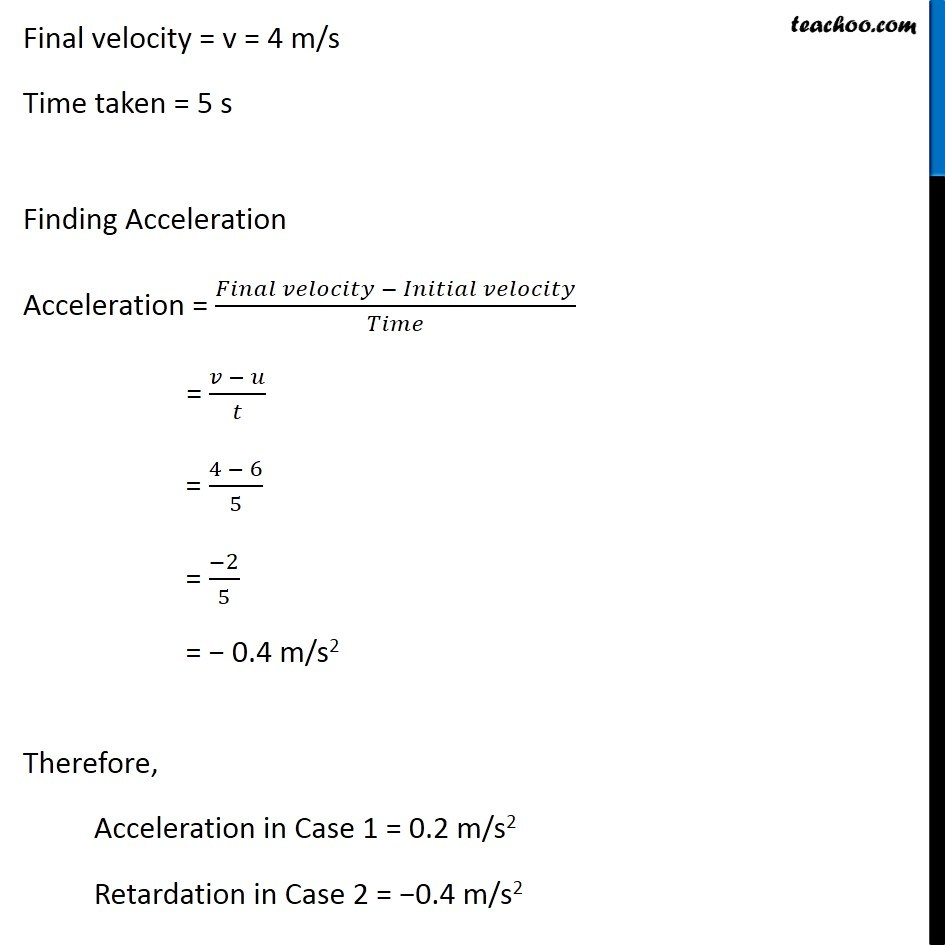Examples from NCERT Book

Class 9
Chapter 8 Class 9 - MotionLearn in your speed, with individual attention - Teachoo Maths 1-on-1 Class

### Transcript

Example 8.4 Starting from a stationary position, Rahul paddles his bicycle to attain a velocity of 6 m s–1 in 30 s. Then he applies brakes such that the velocity of the bicycle comes down to 4 m s-1 in the next 5 s. Calculate the acceleration of the bicycle in both the cases. Case 1 He starts from stationary position, and attains speed of 6 m/s in 30s Since he starts from stationary position Initial velocity = u = 0 m/s Final velocity = v = 6 m/s Time taken = t = 30 s Finding Acceleration Acceleration = (𝐹𝑖𝑛𝑎𝑙 𝑣𝑒𝑙𝑜𝑐𝑖𝑡𝑦 − 𝐼𝑛𝑖𝑡𝑖𝑎𝑙 𝑣𝑒𝑙𝑜𝑐𝑖𝑡𝑦)/𝑇𝑖𝑚𝑒 = (𝑣 − 𝑢)/𝑡 = (6 − 0)/30 = 6/30 = 0.2 m/s2 Case 2 He applies brakes such that the velocity of the bicycle comes down to 4 m s-1 in the next 5 s Here, Final velocity in Case 1 becomes the initial velocity, Initial velocity = u = 6 m/s Final velocity = v = 4 m/s Time taken = 5 s Finding Acceleration Acceleration = (𝐹𝑖𝑛𝑎𝑙 𝑣𝑒𝑙𝑜𝑐𝑖𝑡𝑦 − 𝐼𝑛𝑖𝑡𝑖𝑎𝑙 𝑣𝑒𝑙𝑜𝑐𝑖𝑡𝑦)/𝑇𝑖𝑚𝑒 = (𝑣 − 𝑢)/𝑡 = (4 − 6)/5 = (−2)/5 = − 0.4 m/s2 Therefore, Acceleration in Case 1 = 0.2 m/s2 Retardation in Case 2 = −0.4 m/s2